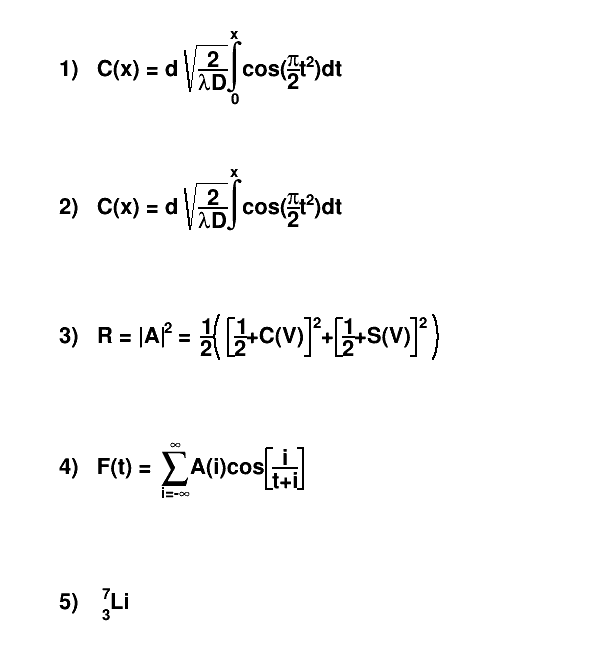# latex¶

This macro draws 5 Latex-style formula in a canvas and prints the canvas as a Postscript file.

Author: Rene Brun
This notebook tutorial was automatically generated with ROOTBOOK-izer from the macro found in the ROOT repository on Monday, August 15, 2022 at 09:36 AM.

In :
TCanvas *c1 = new TCanvas("c1","test",600,700);


write formulas

In :
TLatex l;
l.SetTextAlign(12);
l.SetTextSize(0.04);
#int^{x}_{0}cos(#frac{#pi}{2}t^{2})dt");
#int^{x}cos(#frac{#pi}{2}t^{2})dt");
l.DrawLatex(0.1,0.5,"3)   R = |A|^{2} = #frac{1}{2}#left(#[]{#frac{1}{2}+\
C(V)}^{2}+#[]{#frac{1}{2}+S(V)}^{2}#right)");
l.DrawLatex(0.1,0.3,
"4)   F(t) = #sum_{i=-#infty}^{#infty}A(i)cos#[]{#frac{i}{t+i}}");
l.DrawLatex(0.1,0.1,"5)   {}_{3}^{7}Li");
c1->Print("latex.ps");

Info in <TCanvas::Print>: ps file latex.ps has been created


Draw all canvases

In :
gROOT->GetListOfCanvases()->Draw()